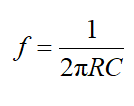# In a Wien bridge oscillator circuit, resistor and capacitor form a series branch and a parallel branch. The value of the resistor is 200 ohm and the value of the capacitor is 10 mF. Determine the frequency of oscillation for the Wien Bridge oscillator circuit.

Question
1 views

In a Wien bridge oscillator circuit, resistor and capacitor form a series branch and a parallel branch. The value of the resistor is 200 ohm and the value of the capacitor is 10 mF. Determine the frequency of oscillation for the Wien Bridge oscillator circuit.

check_circle

Step 1

The frequency of oscillation of a Wien Bridge oscillator is given as...

### Want to see the full answer?

See Solution

#### Want to see this answer and more?

Solutions are written by subject experts who are available 24/7. Questions are typically answered within 1 hour.*

See Solution
*Response times may vary by subject and question.
Tagged in